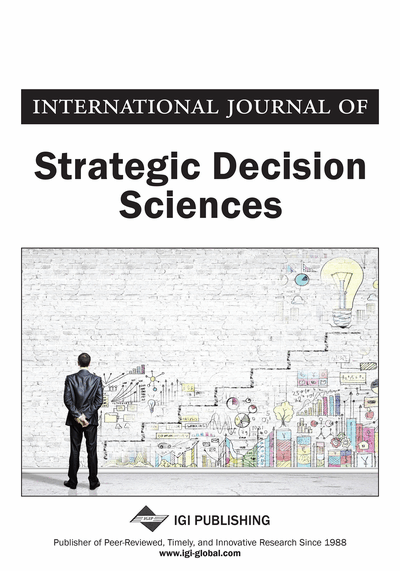# Foundation of Linear Programming: A Managerial Perspective from Solving System of Inequalities to Software Implementation

Hossein Arsham (University of Baltimore, USA)
DOI: 10.4018/jsds.2012070104
Available
\$37.50
No Current Special Offers

## Abstract

The aim and scope of this paper are the infusion of purposeful action by decision makers with an explicit understanding of analytical linear programming (LP) tools in order that the managers will implement strategic results correctly. The advances in computing software have brought LP tools to the desktop for a variety of applications to support managerial decision-making. However, it is already recognized that current LP tools do not answer the managerial questions satisfactorily. For instance, there is a costly difference between the mathematical and managerial interpretations of sensitivity analysis. The LP software packages provide sensitivity results about the optimality of a basis and not about the optimality of the values of the decision variables, and the shadow prices that are of interest to the manager. Society has developed the largest sensitivity region based on “optimal solution,” not that which “preserves the basis” that allows for simultaneous dependent/independent changes. The aim and results of this article are found further in the work.
Article Preview
Top

## 1. Introduction

Linear programming has been a fundamental topic in the development of managerial decision-making. The subject has its origins in the early work of Fourier (1820s) on attempting to solve systems of linear inequalities. However, it has to wait for the invention of the Standard Simplex Method.

There are also many diverse applications, and therefore many specialized solution algorithms have been developed. Hatami-Marbini and Tavana (2011) introduced a new method called the bounded dual simplex method for bounded fuzzy number linear programming problems which is useful for these situations. This algorithm constructs a dual feasible basic solution after obtaining a working basic and then moves towards attaining primal feasibility while maintaining dual feasibility throughout. The use of graph-based LP model is reported by Diaby (2010), Sowlati, Assadi, and Paradi, (2010) extend the LP-based sensitivity analysis to multi-criteria decisionmaking. While Borgonovo and Percoco (2011) applied sensitivity analysis a portfolio problem with budget constraints that allows for simultaneous sensitivity analysis. Khurana and Arora (2012) modified the classical transportation problem to solve a three–dimensional linear trans–shipment problem. Sun (2010) uses linear programming as nonparametric statistical procedures for multiple-class discriminant and classification analysis.

### 1.1. Standard Simplex Method

Since World War II, linear programming (LP) has been used to solve small and large problems in almost all disciplines. The most popular solution algorithm is the Simplex method that is implemented in all LP software packages.

The simplex algorithm can be considered as a sub-gradient directional method, jumping from an initial feasible vertex to a neighboring vertex of feasible region until it arrives at an optimal vertex (if any). To start the algorithm, if it is needed, one must create an equivalent LP problem to satisfy its “Standard form requirement.” When the manager obtains the simplex optimal solution, then the manager has to interpret and to transform the final solution of the algorithm to obtain the optimal solution in terms of the original model, including the variables. This may not be an easy task. Moreover, solving LP problems in which some constraints are in (≥) or (=) form with non-negative right-hand side (RHS) has raised difficulties. One version of the simplex, known as the two-phase method, introduces an artificial objective function, which is the sum of artificial variables (Arsham 1997). Another version adds the penalty terms, which are the sum of artificial variables with very large, positive coefficients. The latter approach is known as the Big-M method (Arsham, 2006, 2007). Understanding the intuitive notion of Standard-form, artificial variables, and Big-M, may require a greater mathematical sophistication from most students/managers. The simplex methods have to iterate through many infeasible vertices to reach an initial feasible vertex.

Using the dual simplex method has its own difficulties. For example, when some coefficients in the objective function are not dual feasible, one must introduce an artificial constraint. Handling equality (=) constraints by the dual simplex method is tedious because of introduction of two new variables for each equality constraint: one extraneous slack variable and one surplus variable. Also, one may not be able to remove some equality (=) constraints by elimination at the outset, as this may violate the non-negativity condition introduced when constructing the “standard form.”

## Complete Article List

Search this Journal:
Reset
Open Access Articles: Forthcoming
Volume 12: 4 Issues (2021): Forthcoming, Available for Pre-Order
Volume 11: 4 Issues (2020): 3 Released, 1 Forthcoming
Volume 10: 4 Issues (2019)
Volume 9: 4 Issues (2018)
Volume 8: 4 Issues (2017)
Volume 7: 4 Issues (2016)
Volume 6: 4 Issues (2015)
Volume 5: 4 Issues (2014)
Volume 4: 4 Issues (2013)
Volume 3: 4 Issues (2012)
Volume 2: 4 Issues (2011)
Volume 1: 4 Issues (2010)
View Complete Journal Contents Listing